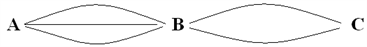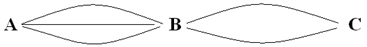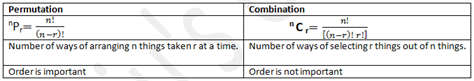# Introduction To Permutation and Combination

Permutations and Combination is one section of the CAT Quantitative Aptitude which needs the least number of formulae and the maximum amount of logic. This topic is asked in almost every competitive exam, so don’t take the risk of avoiding it.  After reading this article you will be familiar with Permutation and Combination.

Definition of Permutation: Permutation is defined as the arrangement of r things that can be done out of total n things.

Formula of Permuation

Permutation is denoted by  nPr   it is equal to  n!/(n-r)!

Defination of Combination: Combination is defined as selection of r things that can be done out of total n things.

Formula of Combination

Combination is denoted  nCit is equal to n!/r!(n-r)!

Practice questions on Permutation and Combination

Fundamental principle of counting

#### a. Product Rule:

If an activity A can be done in “m” ways and an another activity “B” can be done in “n” ways, A and B together can be done in “m x n” ways

Example:

How many ways a person can go from A to C via B?Solution:

A to B in 3 ways

B to C in 2 ways

A to B and B to C in 3 x 2 = 6 ways

If an activity A can be done in “m” ways and an another activity “B” can be done in “n” ways, then A or B together can be done in “m + n” ways

Example:

If the person is at B, how many ways he can go to A or C?Solution:

B to A in 3 ways

B to C in 2 ways

B to A OR

B to C in 3 + 2 = 5 ways

KEYWORDS

AND➜ MULTIPLICATION (Product rule)

### Illustrations:

Question 1) From a pack of cards in how many ways can you select-

a) A king and a queen

b) A king or a queen

Solution:

There are 52 cards in a pack, with 4 kings and 4 queens.

A king can be selected in 4 ways and a queen can be selected in 4 ways. Number of ways of selection of a king AND a queen is 4×4=16 (Product rule)

Number of ways of selection of a king OR a queen 4+4=8 (Addition rule)

The fundamental principle of counting (FPC) is the basic concept of permutation and combination.

2)  In how many ways can 3 people A, B, C be arranged in 2 places?

Solution:

Manually solving we will get 6 cases as given below.

AB BA

AC CA

BC CB

This can be explained using FPC like this

There are two seats __ __. In the first seat A,B or C can sit. i.e., 3 possibilities.

Let A sit in the first place. Thus, in second place either B or C can sit.

The second seat can hence be taken up in 2 ways Together A,B ‘AND’ C can be seated in 3 x 2 = 6 ways.

Thus, the number of arrangement of 3 things at 2 places can be done in 3×2 ways.

3) In how many ways can 4 people be seated in 3 chairs?

Solution:

First seat can be filled in 4 ways.

Second seat can be filled in 3 ways.

Third seat can be filled in 2 ways.

So all together 4 people can be arranged in 3 seats in 4x3x2 = 24 ways.

Generalizing; arrangement of n things at r places can be done in [n(n-1)(n-2)…(n-r+1)] ways.

This arrangement of n things at r places is denoted by nPr .

∴ nPr = n(n-1)(n-2)…(n-r+1) = n!/(n-r)!

It should be noted that in all these examples, the order of arrangement is important.

In other words, AB and BA are counted as 2 different cases. An arrangement where the order is important is called permutation

Now let us see one case where the order is not important.

Example:

4) How many doubles teams can be selected from 3 persons A, B, C?

Solution:

Let’s take a doubles team AB. Change the order, i.e. to BA. Notice that both the teams are one and the same.

So here the order is not important. Hence, it’s a case of combination. Here one can select the team in 3 ways

AB

AC

BC

If the order is important, then the total number of arrangements can be done in nPr = 3P2 ways.

We will get the 6 cases as:

AB BA

AC CA

BC CB

But in this particular question every 2! (because A,B can be arranged in 2! Ways) cases will be counted as only one

case, i.e AB and BA is one and the same team.

So the number of selections of 2 things from 3 things can be done in = 3(P2 )/2!

Similarly, the number of selections of 3 things from 4 things can be done in =4P3/3!

Generalizing: the number of selections of r things from n things can be done in = nPr/r! ways.

The number of selections of r things from n different things is denoted by nCr,

which is what we learn as “Combination”.

∴ nCr = nPr/r!=n!/[(n-r)! r!]

Thus, you can understand now, that wherever the arrangement is necessary apart from selection,

we used Permutation and when we just need to select without arranging, we use Combination.

To make the point even clearer.

Let us look at one more example:

5) In how many ways can you select a President and a Vice-President from 3 people?

Is it a case of permutation or combination?

In the above question, since the word “select” appears, it may tempt you to consider combination.

So let’s take a case where A is the President and B is the Vice-President. Now change the order,

i.e. B is President and A Vice-President. Are both the cases same? No, they are not! We see that both the cases are different.

The order is important. Hence it is a case of permutation.

#### Difference between Permutation and Combination:The relation between permutation and combination

∴ nPr = nCr x r!

The permutation of ‘n’ things taking ‘r’ at a time will involve the following steps:

i) Select ‘r’ things out of total ‘n’ things, which can be done in nCr ways.

ii) Arrange those ‘r’ things among themselves which can be done in r! ways.

nPr is nothing but, selecting r things out of n things (in nCr ways) and then arranging it among themselves.

This is why every permutation is selection followed by the arrangement of the selected things among themselves.

If it is only selection where the arrangement is not important, then we use the combination (nCr) only.

To learn more such CAT exam topics easily, stay tuned with Byju’s. Also, get the latest syllabus and pattern for the CAT along with various video lessons to learn more effectively.